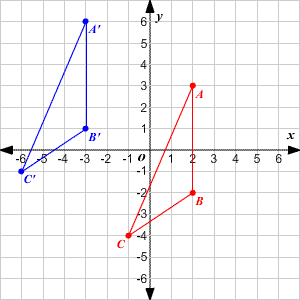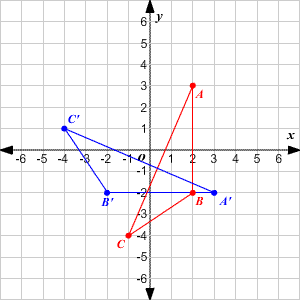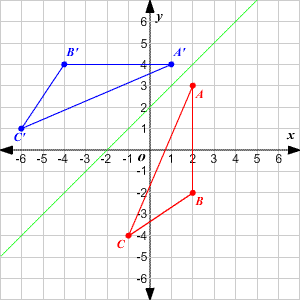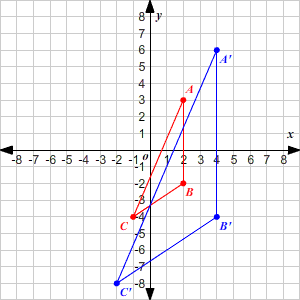# Transformations

There are three kinds of isometric transformations of $2$ -dimensional shapes: translations, rotations, and reflections. ( Isometric means that the transformation doesn't change the size or shape of the figure.) A fourth type of transformation, a dilation , is not isometric: it preserves the shape of the figure but not its size.

## Translations

A translation is a sliding of a figure. For example, in the figure below, triangle $ABC$ is translated $5$ units to the left and $3$ units up to get the image triangle ${A}^{\text{'}}{B}^{\text{'}}{C}^{\text{'}}$ .This translation can be described in coordinate notation as $\left(x,y\right)\to \left(x-5,y+3\right)$ .

## Rotations

A second type of transformation is the rotation . The figure below shows triangle $ABC$ rotated $90°$ clockwise about the origin.This rotation can be described in coordinate notation as $\left(x,y\right)\to \left(y,-x\right)$ . (You can check that this works by plugging in the coordinates $\left(x,y\right)$ of each vertex.)

## Reflections

A third type of transformation is the reflection . The figure below shows triangle $ABC$ reflected across the line $y=x+2$ .This reflection can be described in coordinate notation as $\left(x,y\right)\to \left(y-2,x+2\right)$ . (Again, you can check this by plugging in the coordinates of each vertex.)

## Dilations

A dilation is a transformation which preserves the shape and orientation of the figure, but changes its size. The scale factor of a dilation is the factor by which each linear measure of the figure (for example, a side length) is multiplied.

The figure below shows a dilation with scale factor $2$ , centered at the origin.This dilation can be described in coordinate notation as $\left(x,y\right)\to \left(2x,2y\right)$ . (Again, you can check this by plugging in the coordinates of each vertex.)